# Physics - Electromagnetism - Electromagnetic Wave Introduction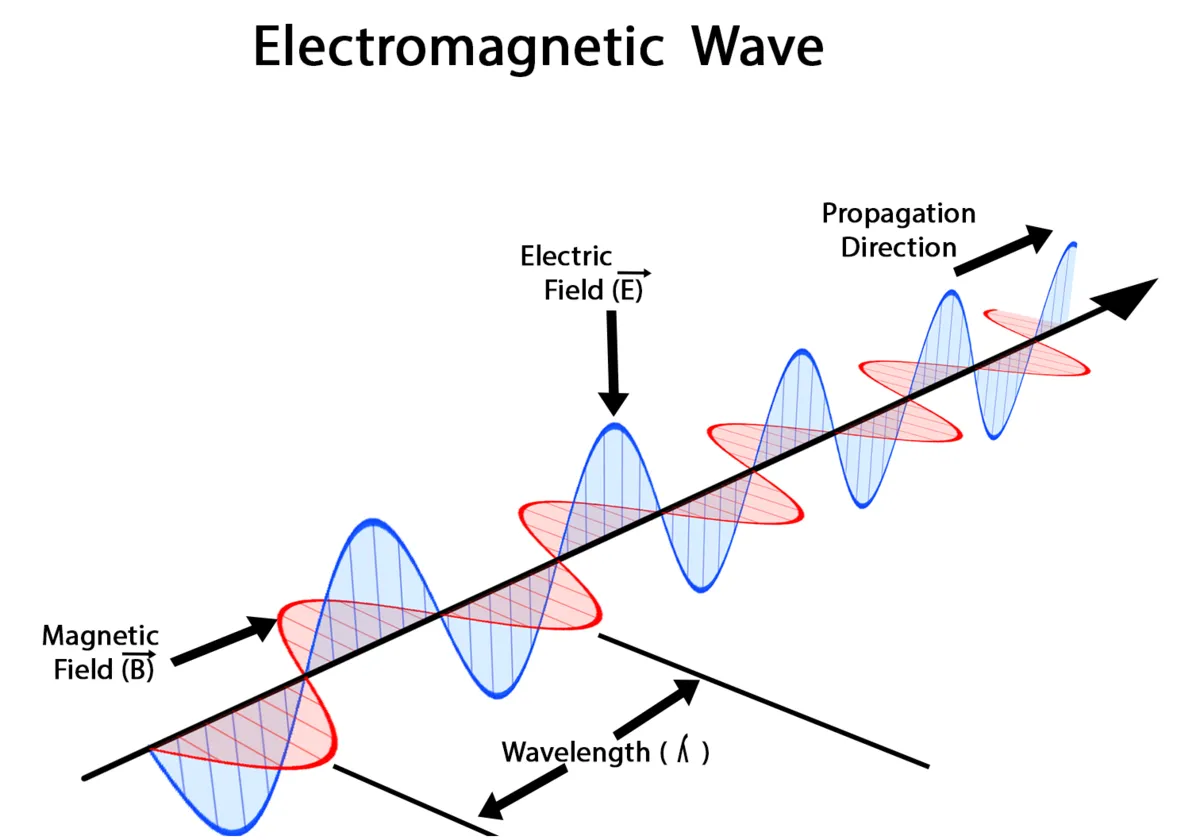Image source:## Introduction

Hello it's a me again Drifter Programming! Today we continue with Electromagnetism to get into Electromagnetic Waves. We will get into a small Introduction that will basically describe what they are, and afterwards also talk about their velocity and also the "famous" wave equation. So, without further do, let's get started!

## Electromagnetic Waves

Let's start out with what electromagnetic waves are. Electromagnetic waves or EM waves are the result of the vibrations between an electric field and a magnetic field. This means that they are composed by oscillating magnetic and electric fields. The magnetic field and electric field of course oscillate in-phase. Such waves are formed when an electric field interacts with a magnetic field. The two fields, electric and magnetic, are perpendicular to each other and also perpendicular to the direction that the EM wave propagates to. They cannot be deflected by an electric field or by an magnetic field. Electromagnetic waves travel at the speed of light (3x10^8 m/s) in vacuum and can travel through anything. By that I mean that they can travel through a vacuum, through air (that contains molecules) or even a solid material. The interesting thing is that they don't need a medium to travel from one place to another. Mechanical waves (sound or water waves) need a medium to travel with. We also say that EM waves are "transverse" waves, cause they are measured by their amplitude (height) and wavelength (distance between the highest/lowest points of two consecutive waves). The highest point is known as 'crest', whilst the lowest point is known as 'trough'.

From all that we understand that the transmission of energy through a vacuum (or using no medium) can be accomplished by EM waves. Those waves are often called electromagnetic radiation, light or photons! Yes, you heard right. All the light that we see in our daily life, including the light from your screen right now, is actually an Electromagnetic wave of specific frequency! That's why we split the Electromagnetic waves by their frequencies, building the so called electromagnetic spectrum, that we will cover separately in later on article. Remember that light is not always acting like a wave, but sometimes also as a particle. Light has "two" natures at-once, depending on the way we approach it.

We use them in lots of applications including:

• the transmission of long/short/FM wavelength radio waves
• in TV/telephone/wireless signals
• when heating food in a micro oven, using microwaves
• as heat sensors using infrared radiation (IR)
• sterilisation of equipment and more using ultraviolet light (UV)
• to "see' when talking about the visible light (VIS)
• bone "photography" using X-Rays

Note that all those including some other types like gamma ray, cosmic rays are the categorizations of EM waves. More about this spectrum will be covered in a coming up article...

Being a composition of electric and magnetic fields we can use Maxwell's equations to analyze their behavior! You might rememeber that Maxwell's equation describe the relationship between electricity and magnetism in just four equations. When inside of a vacuum (region without charges or currents) these four equation can be reduced into:Something last that is worth noting is that the unit of frequency Hertz (Hz) got it's name from Heinrich Hertzt that proved the existence of radio waves. The number of crests (high points) that pass a given point in space within one second is described as the frequency of the wave. Simiarly, we define the number of some physical quantity per second again as the frequency of some other quantity.

So, let's summarize everything that we told till now:

• EM waves are the caused by changing electric and magnetic fields, that get linked together
• They don't require a medium to travel, which means that they can travel through a vacuum of space
• Light or photons are electromagnetic waves
• They have three main properties: frequency, wavelength, amplitude
• They are categorized depending on their frequency AND
• Can be analyzed mathematically using Maxwell's equation

## Velocity

Let's proof why their velocity is c = 3 x 10^8 m/s.

Consider a plane electromagnetic wave propagating along the z-axis. According two Maxwell's laws, the electric and magnetic fields can be described as: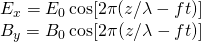You can clearly see that they are perpendicular to each other and also to the propagating direction! Those fields are periodic in time and space. The oscillating frequency of the fields is f.

The equation for a wave crest is: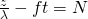where N is a n integer.

The distance between successive wave crests is λ, which clearly shows us that λ is representing the wavelength. In time interval dt, the Nth wave crest will move a distance of dz = λfdt (along the z-axis of course). The velocity is of course v = dz/dt, which shows us that:An equation that combined the frequency and wavelength together! There of course is no constraint on the possible frequency or wavelength of EM waves.

In previous articles we saw that:where:

• the first one describes the relationship between the maximum amplitudes of the two fields
• the second one gives us the "fixed" propagation velocity of EM waves, cause μ and ε are constants

We know that these constant have the value:
From that the light-speed comes out ~3 x 10^8 m/s!

It's remarkable that the experimental proof and theoretical predition are so close! Seeing that electromagnetic waves travel with the speed of light (c) we can clearly see why light is a form of EM wave.

## Wave equation

Electric waves travelling through space can be described by a so called wave equation: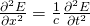The same can also be done for magnetic fields...

As a plane wave solution the electric and magnetic field are described using sinus functions (or cosinus function the same way as we used them in our proof) as:You can see that we now used the angular velocity instead...

### REFERENCES:

Mathematical equations that I had in this post where drawn using quicklatex!

## Previous articles of the Electromagnetism series

Here we only have the previous two "chapters"....

### Mutual and Self Induction:

Mutual Inductance -> Mutual Inductance, applications

Self Induction -> Self Induction, Lenz's law, Inductance of a Coil

Magnetic Energy Density -> Energy stored in a magnetic field (or inductor), Magnetic energy density, Coaxial Cable Inductance Example

R-L circuits -> R-L circuit energizing, de-energizing, Characteristic time constant

L-C circuits -> L-C circuit, oscillations, energy cases, applications

R-L-C circuits -> R-L-C circuit, oscillation, applications

Mutual and Self Induction exercises -> examples all around Mutual and Self Induction

### Alternating current:

Getting into Alternating current -> Phasor diagrams, Alternating current, Average (RMS) current and voltage, Differences, Advantages/Disadvantages

Electric Reactance in AC circuits -> Resistors, Inductor and Capacitor Reactance in AC circuits

Series R-L-C circuits Impedance -> Series R-L-C circuits, Phasor Diagrams, Impedance

Power in AC circuits -> DC Resistive circuit, Power in AC circuits (resistive, reactive), Power Factor

Resonance in Series R-L-C circuits -> Series R-L-C circuit, Series Resonance

Resonance in Parallel R-L-C circuits -> Parallel R-L-C circuit, Parallel Resonance

AC Transformers -> How they work, Configurations, Types, Voltage and Current Transformer

Alternating current exercises -> examples all around Alternating current

## Final words | Next time

And this is actually it for today's post and I hope that you enjoyed it! Next time we will continue on with Sine waves.Keep on drifting!

H2
H3
H4
3 columns
2 columns
1 column